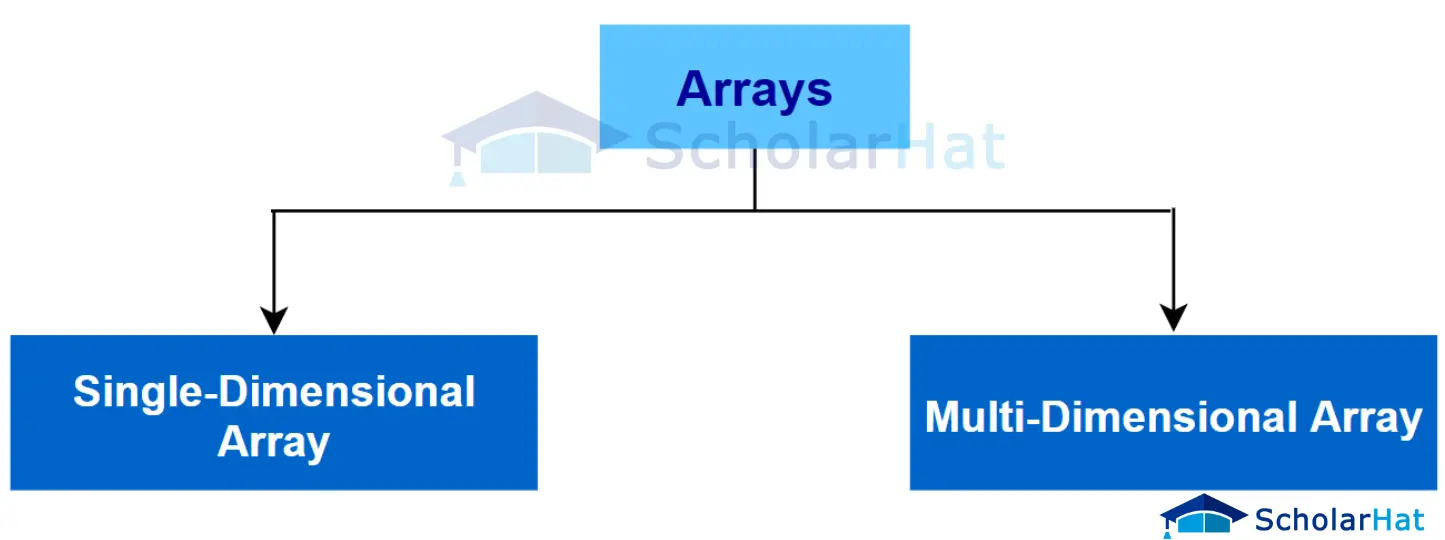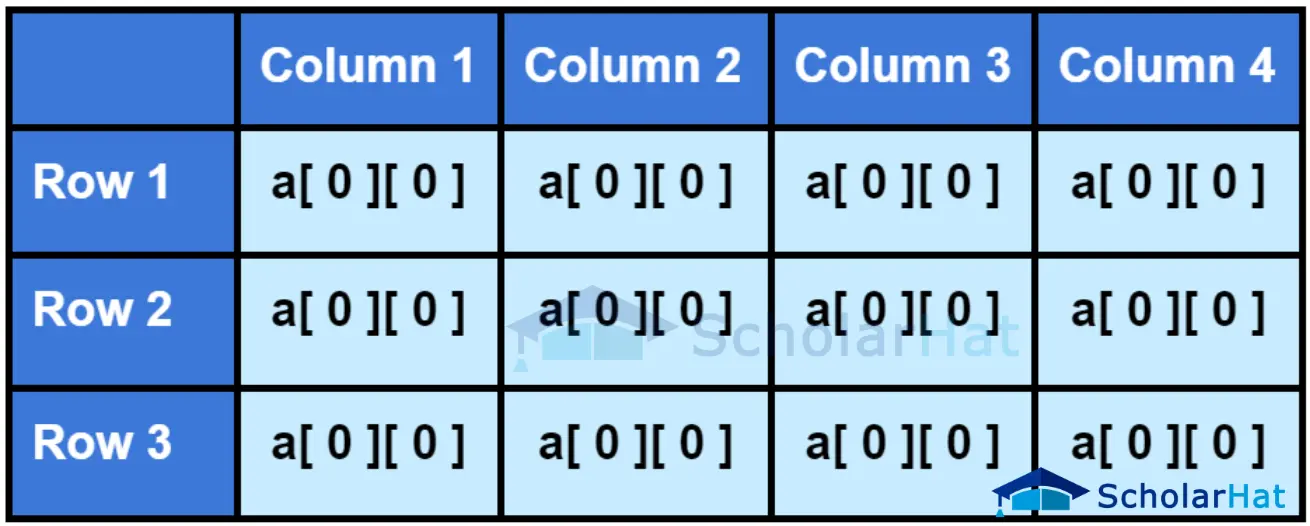# Java Arrays: Single and Multi-Dimensional Arrays

22 Sep 2023
Beginner
7.7K Views

## Java Arrays: An Overview

In this article, the implementation of arrays in Java, Java Arrays, array in Java example, java array methods, and Arrays in Java will be discussed. This article aims to help readers learn Java and highlights the advantages and disadvantages of various types of Java Arrays.

## What is an Array?

An array is a type of data structure that is used to store the elements of a particular type. For example, an integer array will hold only the value of any integer, but a character array will hold the characters only. The structure of an array is linear and holds similar elements in "contiguous memory addresses". In Java, not only array but also exception handling is essential to manage and deal with unexpected errors that may occur during the execution of a program which helps the process of execution easy.

## Pros and Cons of Using Array

### Advantages of using Java arrays

• Arrays help to increase code optimization
• It helps in ordering and indexing data structure to store a particular type of element
• It can also store variables and objects of class in java
• Every element of an array is held in a definite memory location

### Disadvantages of using Java arrays

• An array can only hold the same type of elements
• Removing and adding elements in very tough

## Types of Array in Java

There are two types of arrays in Java:

1. Single dimensional array in Java
2. Multi dimensional array in Java### Single Dimensional Array in Java

• Single Dimensional Array in Java is basically a linear array that allows its user to store multiple values of the same data type.
• It's a collection of data that stores elements of the same type in a sequentially allocated space in memory.
• Single-dimensional arrays can be utilized to store both simple and complex data types, anything from strings, integers, and booleans to custom-made classes depending on the user's requirements.
• In memory, a one or single-dimensional array holds the data in a linear list.
• The index of the memory runs from the array size of 0 to -1.
• Single-Dimensional Arrays are initialized just like regular variables, but instead of assigning a single value the programmers can pass several values separated by commas or define an array with static size.
• Single-Dimensional Arrays are one of the handy tools contained within Java.
• They are useful for organizing data quickly and efficiently as well as giving us access to stored data using looping structures.

### Multi Dimensional Array in Java

• Multi Dimensional Array in Java is basically an array of arrays, which means that it is an array object that has multiple dimensions.
• Multi-Dimensional Arrays are useful when dealing with a large amount of data since they give the ability to store and access data from a single variable but with multiple levels of hierarchy.
• This multi-dimensional array can be expanded to a certain number of dimensions such as two dimensions, three dimensions, etc.
• Multi-Dimensional Arrays in Java can hold any type of item, i.e. two-dimensional arrays can hold int values or objects such as strings and objects.
• Multi-dimensional arrays also have several methods available to help search and arrange the data within the array, making them very flexible and efficient when dealing with complex tasks.## How to Declare and Initialize an Array in Java?

#### The syntax for one-dimensional array in Java:

``arrayName = new DataTye[length 1][length 2]....[length N];(OR)DataType[1st Dimension][2nd Dimension]....[Nth Dimension] arrayName = new DataTye[length 1][length 2]....[length N];``

#### Example

``````//declaration
int iArray1[] = new int;

//initialization
iArray1 = 32;
iArray1 = 13;
iArray1 = 56;
iArray1 = 78;``````

In this example, a size-4 integer array named "iArray1" is declared and initialized with predetermined values, with each element's index denoting a different integer value.

#### The syntax for multi-dimensional array in Java:

``````Datatype [][]Array_Name = new Datatype[row_size][col_size(optional)]; (OR)

Datatype Array_Name[] = [{Value separated by comma},{Value separated by comma},....}];
``````

#### Example

``````//declaration
int matrix[] = new int;

//initialization
matrix = 1;
matrix = 2;
matrix = 3;
matrix = 4;``````

This example declares and initializes a 2x2 integer matrix (a 2-dimensional array), where each member at a given row and column index corresponds to a different integer value.

## Accessing Elements of Array in Java

There are three types of Accessing Element:

2. Accessing elements using a loop
3. Accessing elements using for-each loop

This access method gives the user the authority to access the exact element directly of an array by using an index number.

#### Syntax

``Array_Name[index]``

#### Example

``````//directly accessing the second element of the array using the index number
iArray;``````

The value stored at index 1 of the array is retrieved in this example by directly accessing the second member of the array 'iArray' using the index number.

### Accessing elements using for loop

In this access method, any particular array can be accessed by using iteration statements such as,for loop.

#### Example

``````class MainDemo {

public static void main(String[] args) {

// create an array
int[] numbers = {1, 4, 5, 8, 7};

// using for loop go through the array
for(int i = 0; i < numbers.length; i++) {

System.out.println(age[i]);

}
}
}``````

This Java program creates the integer array "numbers" with predetermined values and then uses a "for" loop to traverse through the array, printing each element one at a time. However, there is a mistake in the code; to print the contents of the 'numbers' array, the correct syntax is 'System.out.println(age[i]);' rather than 'System.out.println(numbers[i]);'.

### Accessing elements using for-each loop

In this method, the for-each loop can also be used for accessing the elements of the array

#### Example

``````class MainDemo {

public static void main(String[] args) {

// create an array
int[] numbers = {1, 2, 4, 6, 8};

// using for-each loop to go through each element
for(int i : numbers) {

System.out.println(i);

}
}
}``````

This Java program populates the integer array "numbers" with particular values, then iterates through each element of the array using a for-each loop, writing each element on a separate line.

## Assigning an Array Reference to Another Array

An array can be used as a reference type of variable and can be used to assign an already existing array.

#### Example

``````class MainDemo
{

public static void main(String[] args)

{
// create an array

int[] arr = {1, 2, 4, 6, 8};
int[] arr1;
arr1 = arr; //assigning arr array reference to arr1

System.out.println(arr1);

}
}``````

This Java code creates the integer array 'arr' with the specified values. In order for 'arr' and 'arr1' to point to the same array in memory, another integer array 'arr' is declared and given the reference of 'arr'. As a result, 'arr1' outputs the value 6, which is located at index 3 of the shared array.

#### Output

``````Java -cp /tmp/ovXXlntsc7 MainDemo
6
``````

## FAQs

### 1. What are arrays in Java?

In Java, arrays are types of data structures that keep a fixed-length collection of identically sized elements.

### 2. What are the types of arrays in Java?

Primitive arrays (such as int[] and double[]) & reference arrays (such as String[]) are the two types of arrays used in Java.

### 3. How to Initialize an Array in Java?

When declaring an array in Java, you can initialize it using an array initializer or by setting values to individual elements after declaration.

### 4. How to declare arrays in Java?

Java requires the data type, the array name, and optional square brackets before declaring an array. Examples include int[] numbers;

### 5. What is a loop in Java?

In Java, a loop is a control structure used for executing a block of code repeatedly. Common types of loops include for, while, and do-while loops.

### 6. What are the basic concepts of an array?

Basic concepts of arrays include elements, indexes, and length. Elements are the values stored in the array, indexes are the positions of elements, and length is the number of elements in the array.

### 7. Why do we need an array?

Arrays are used to store and manipulate collections of data efficiently, enabling easy access, modification, and traversal of elements, making them essential for many programming tasks.

##### Summary

This article provides an insightful overview of the definition, pros, and cons of arrays, making it a valuable resource for individuals undergoing Java Certification Training. Moreover, it thoroughly covers the concepts of single-dimensional arrays and multi-dimensional arrays in Java, further enhancing your understanding of array manipulation. Additionally, it includes detailed explanations of the methods used for array declaration and initialization. If you want to learn Java, it also includes the methods of declaration and initialization of an array.

Similar Articles